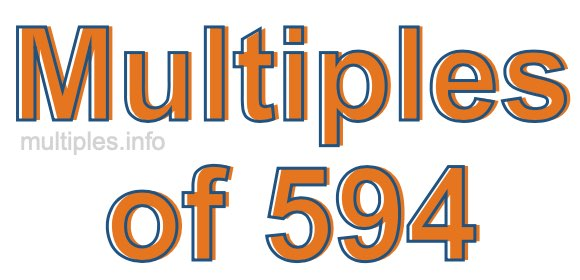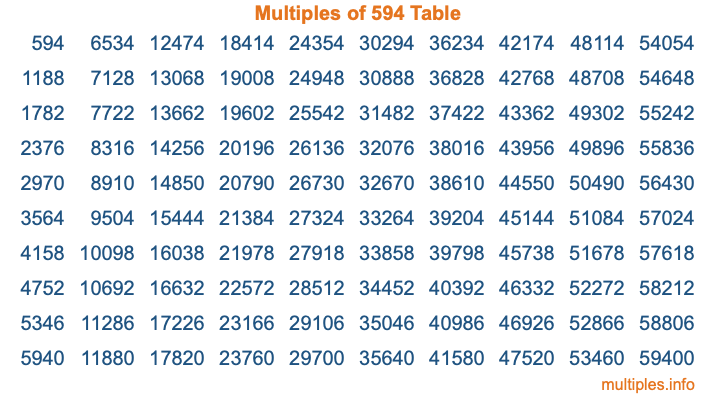Multiples of 594Welcome to the Multiples of 594 page. Here we will first teach you everything you will ever need to know about the multiples of 594, and then give you a study guide summary of everything we taught you to make sure you remember it all. Use this page to look up facts and learn information about the multiples of 594. This page will make you a multiples of five hundred ninety-four expert!

Definition of Multiples of 594
Multiples of 594 are all the numbers that when divided by 594 equal an integer. Each of the multiples of 594 are called a multiple. A multiple of 594 is created by multiplying 594 by an integer.

Therefore, to create a list of multiples of 594, you start with 1 multiplied by 594, then 2 multiplied by 594, then 3 multiplied by 594, and so on for as long as you want. Thus, the list of the first five multiples of 594 is 594, 1188, 1782, 2376, and 2970. To see a larger list of multiples of 594, see the printable image of Multiples of 594 further down on this page. We also have a category where you can choose any nth multiple of 594.

Multiples of 594 Checker
The Multiples of 594 Checker below checks to see if any number of your choice is a multiple of 594. In other words, it checks to see if there is any number (integer) that when multiplied by 594 will equal your number. To do that, we divide your number by 594. If the the quotient is an integer, then your number is a multiple of 594.

Is  a multiple of 594?

Least Common Multiple of 594 and ...
A Least Common Multiple (LCM) is the lowest multiple that two or more numbers have in common. This is also called the smallest common multiple or lowest common multiple and is useful to know when you are adding our subtracting fractions. Enter one or more numbers below (594 is already entered) to find the LCM.

Check out our LCM Calculator if you need more details about the Least Common Multiple or if you need the LCM for different numbers for adding and subtraction fractions.

nth Multiple of 594
As we stated above, 594 is the first multiple of 594, 1188 is the second multiple of 594, 1782 is the third multiple of 594, and so on. Enter a number below to find the nth multiple of 594.

th multiple of 594

Multiples of 594 vs Factors of 594
594 is a multiple of 594 and a factor of 594, but that is where the similarities end. All postive multiples of 594 are 594 or greater than 594. All positive factors of 594 are 594 or less than 594.

Below is the beginning list of multiples of 594 and the factors of 594 so you can compare:

Multiples of 594: 594, 1188, 1782, 2376, 2970, etc.

Factors of 594: 1, 2, 3, 6, 9, 11, 18, 22, 27, 33, 54, 66, 99, 198, 297, 594

As you can see, the multiples of 594 are all the numbers that you can divide by 594 to get a whole number. The factors of 594, on the other hand, are all the whole numbers that you can multiply by another whole number to get 594.

It's also interesting to note that if a number (x) is a factor of 594, then 594 will also be a multiple of that number (x).

Multiples of 594 vs Divisors of 594
The divisors of 594 are all the integers that 594 can be divided by evenly. Below is a list of the divisors of 594.

Divisors of 594: 1, 2, 3, 6, 9, 11, 18, 22, 27, 33, 54, 66, 99, 198, 297, 594

The interesting thing to note here is that if you take any multiple of 594 and divide it by a divisor of 594, you will see that the quotient is an integer.

Multiples of 594 Table
Below is an image of the first 100 multiples of 594 in a table. The table is in chronological order, column by column. The first column has the first ten multiples of 594, the second column has the next ten multiples of 594, and so on.The Multiples of 594 Table is also referred to as the 594 Times Table or Times Table of 594. You are welcome to print out our table for your studies.

Negative Multiples of 594
Although not often discussed or needed in math, it is worth mentioning that you can make a list of negative multiples of 594 by multiplying 594 by -1, then by -2, then by -3, and so on, to get the following list of negative multiples of 594:

-594, -1188, -1782, -2376, -2970, etc.

Multiples of 594 Summary
Below is a summary of important Multiples of 594 facts that we have discussed on this page. To retain the knowledge on this page, we recommend that you read through the summary and explain to yourself or a study partner why they hold true.

There are an infinite number of multiples of 594.

A multiple of 594 divided by 594 will equal a whole number.

594 divided by a factor of 594 equals a divisor of 594.

The nth multiple of 594 is n times 594.

The largest factor of 594 is equal to the first positive multiple of 594.

594 is a multiple of every factor of 594.

594 is a multiple of 594.

A multiple of 594 divided by a divisor of 594 equals an integer.

594 divided by a divisor of 594 equals a factor of 594.

Any integer times 594 will equal a multiple of 594.

Multiples of a Number
Here you can get the multiples of another number, all with the same attention to detail as we did for multiples of 594 on this page.

Multiples of
Multiples of 595
Did you find our page about multiples of five hundred ninety-four educational? Do you want more knowledge? Check out the multiples of the next number on our list!